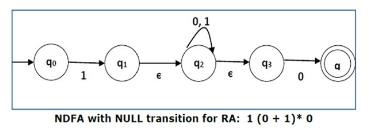# Convert RE 1(0+1)*0 into equivalent DFA.

To convert the regular expression to Finite Automata (FA) we can use the Subset method.

Subset method is used to obtain FA from the given regular expression (RE).

• Step 1 − Construct a Transition diagram for a given RE using Non-deterministic finite automata (NFA) with ε moves.
• Step 2 − Convert NFA with ε to NFA without ε.
• Step 3 − Convert the NFA to equivalent DFA.

We will divide the given expression into three parts as follows −

“1” ,”(0+1)*, and “0”

NFA with Epsilon transition is as follows −Now, we will remove the epsilon transition.

After removing, the transition diagram is given below −